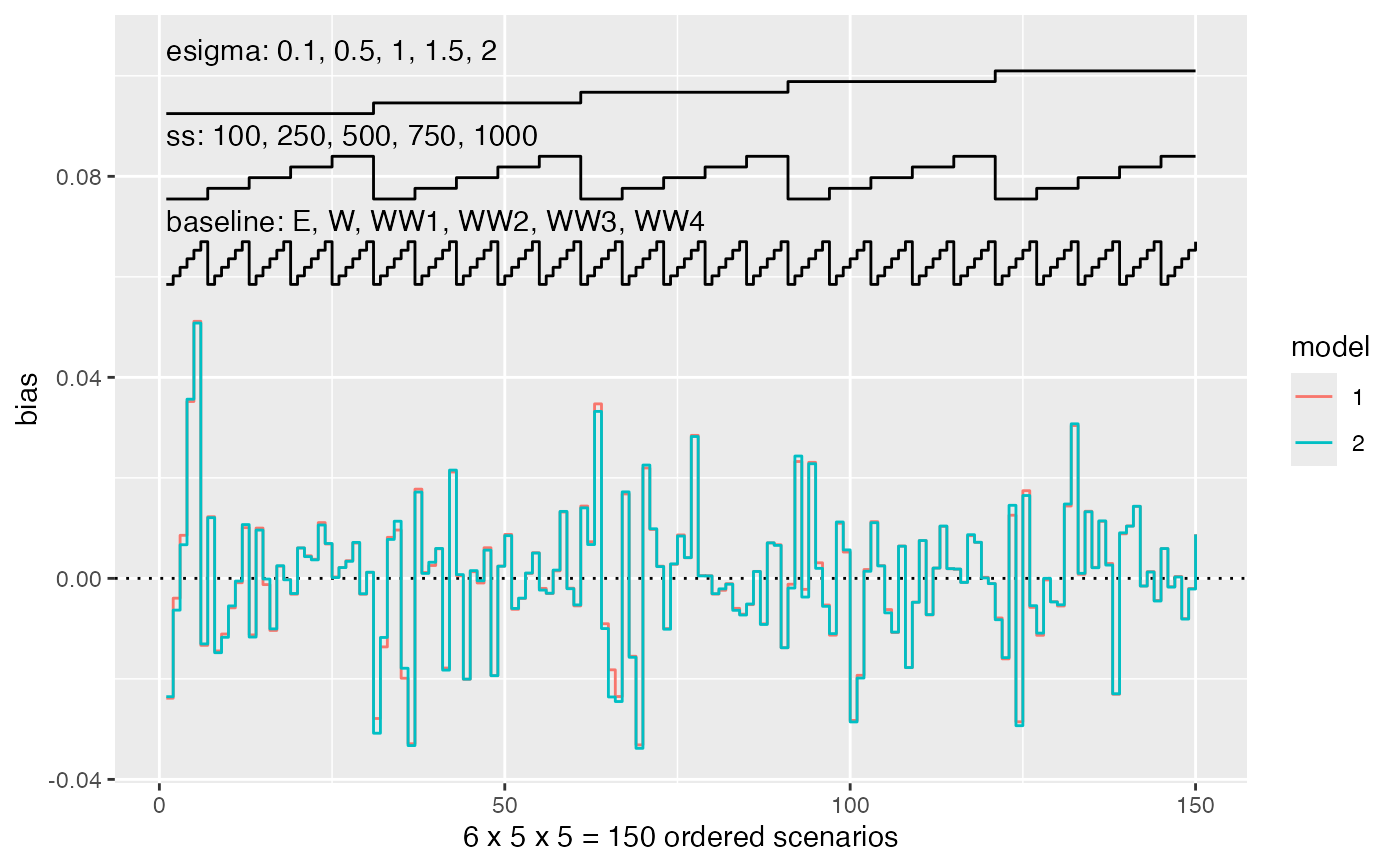autoplot can produce a series of plot to summarise results of simulation studies. See vignette("plotting", package = "rsimsum") for further details.

# S3 method for simsum
autoplot(
object,
type = "forest",
stats = "bias",
target = NULL,
fitted = TRUE,
scales = "fixed",
top = TRUE,
density.legend = TRUE,
zoom = 1,
...
)

## Arguments

object An object of class simsum. The type of the plot to be produced. Possible choices are: forest, lolly, zip, est, se, est_ba, se_ba, est_ridge, se_ridge, est_density, se_density, est_hex, se_hex, heat, nlp, with forest being the default. Summary statistic to plot, defaults to bias. See summary.simsum() for further details on supported summary statistics. Target of summary statistic, e.g. 0 for bias. Defaults to NULL, in which case target will be inferred. Superimpose a fitted regression line, useful when type = (est, se, est_ba, se_ba, est_density, se_density, est_hex, se_hex). Defaults to TRUE. Should scales be fixed (fixed, the default), free (free), or free in one dimension (free_x, free_y)? Should the legend for a nested loop plot be on the top side of the plot? Defaults to TRUE. Should the legend for density and hexbin plots be included? Defaults to TRUE. A numeric value between 0 and 1 signalling that a zip plot should zoom on the top x% of the plot (to ease interpretation). Defaults to 1, where the whole zip plot is displayed. Not used.

## Value

A ggplot object.

## Examples

data("MIsim", package = "rsimsum")
s <- rsimsum::simsum(
data = MIsim, estvarname = "b", true = 0.5, se = "se",
methodvar = "method", x = TRUE
)#> 'ref' method was not specified, CC set as the reference
library(ggplot2)
autoplot(s)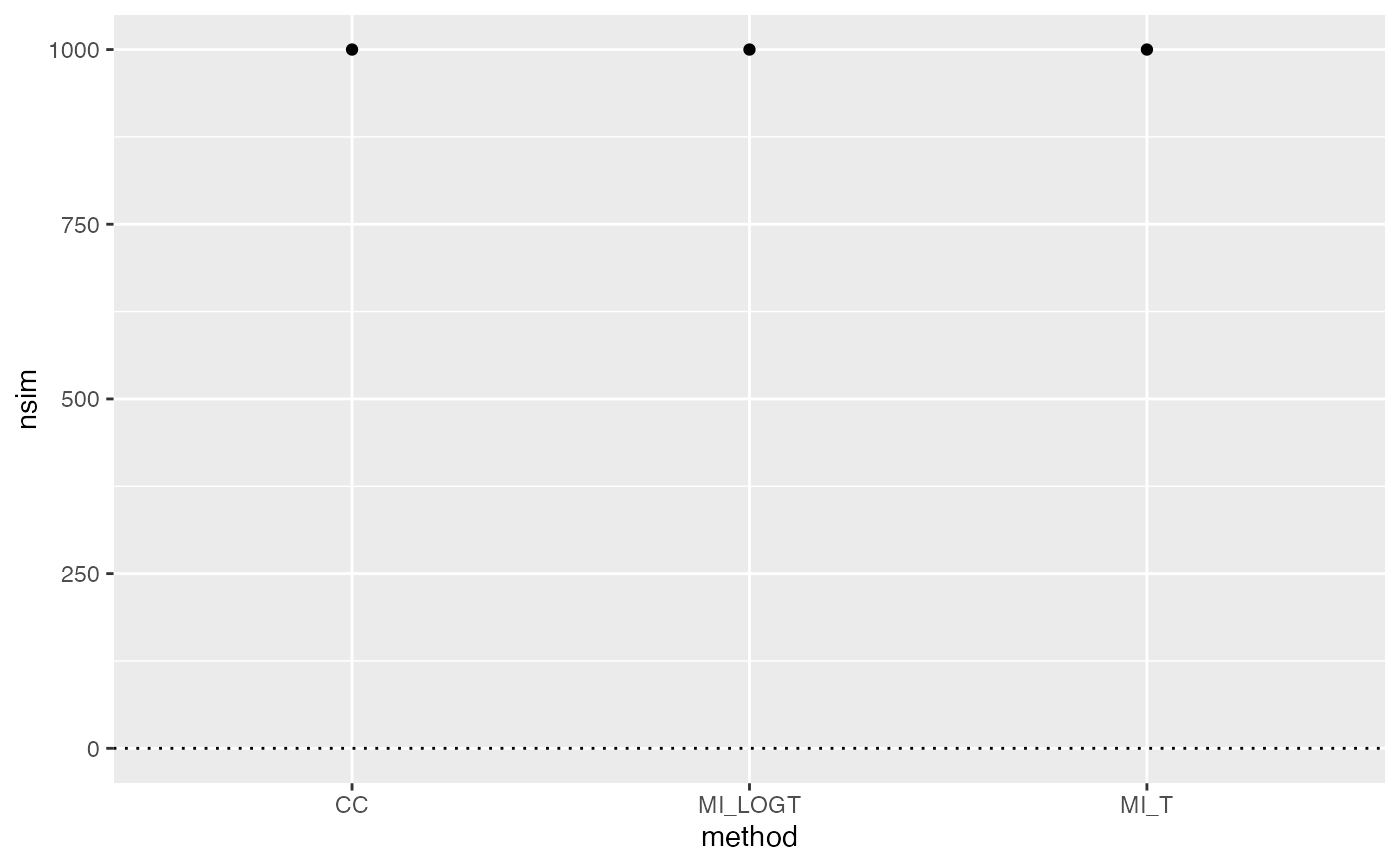autoplot(s, type = "lolly")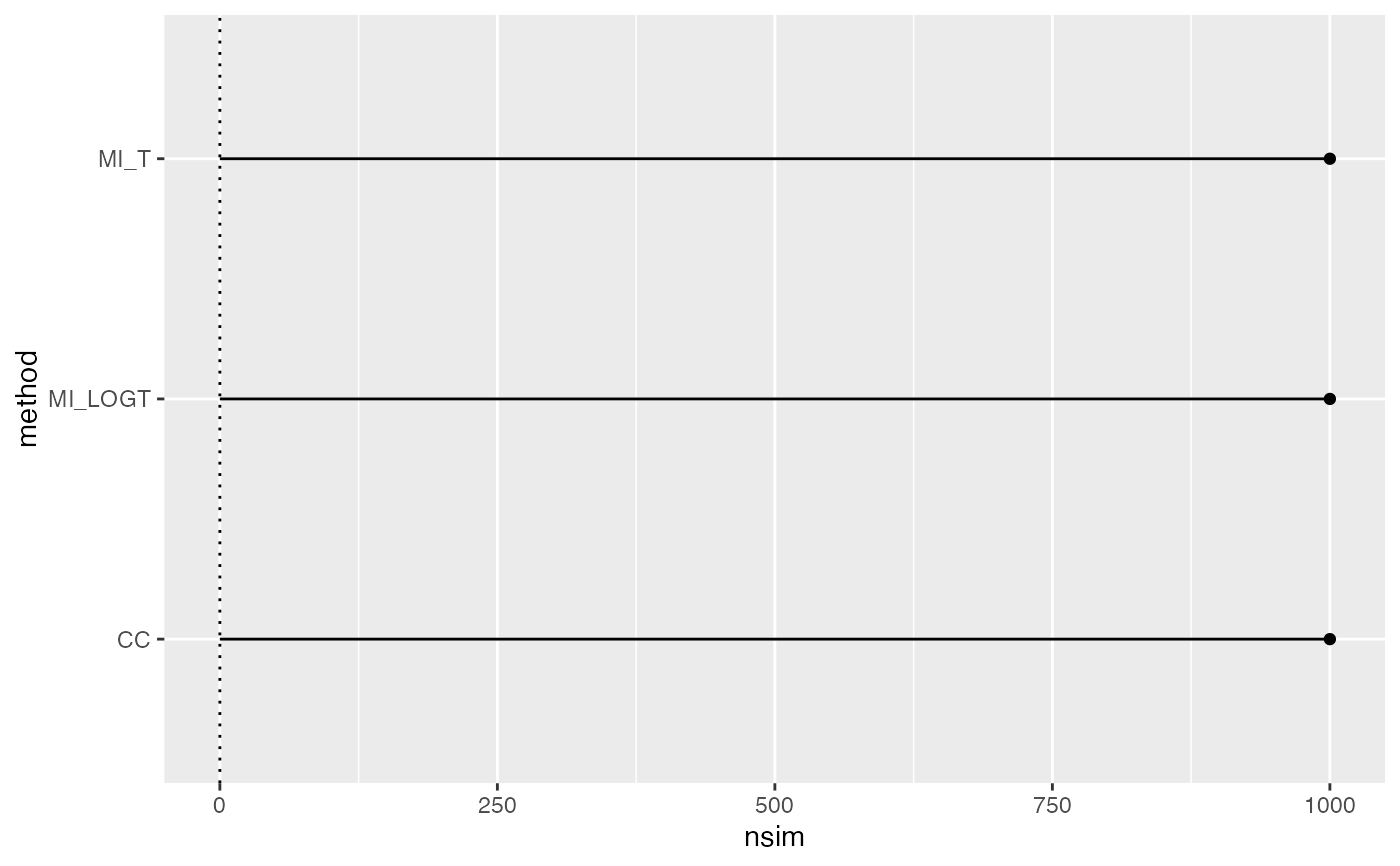autoplot(s, type = "est_hex")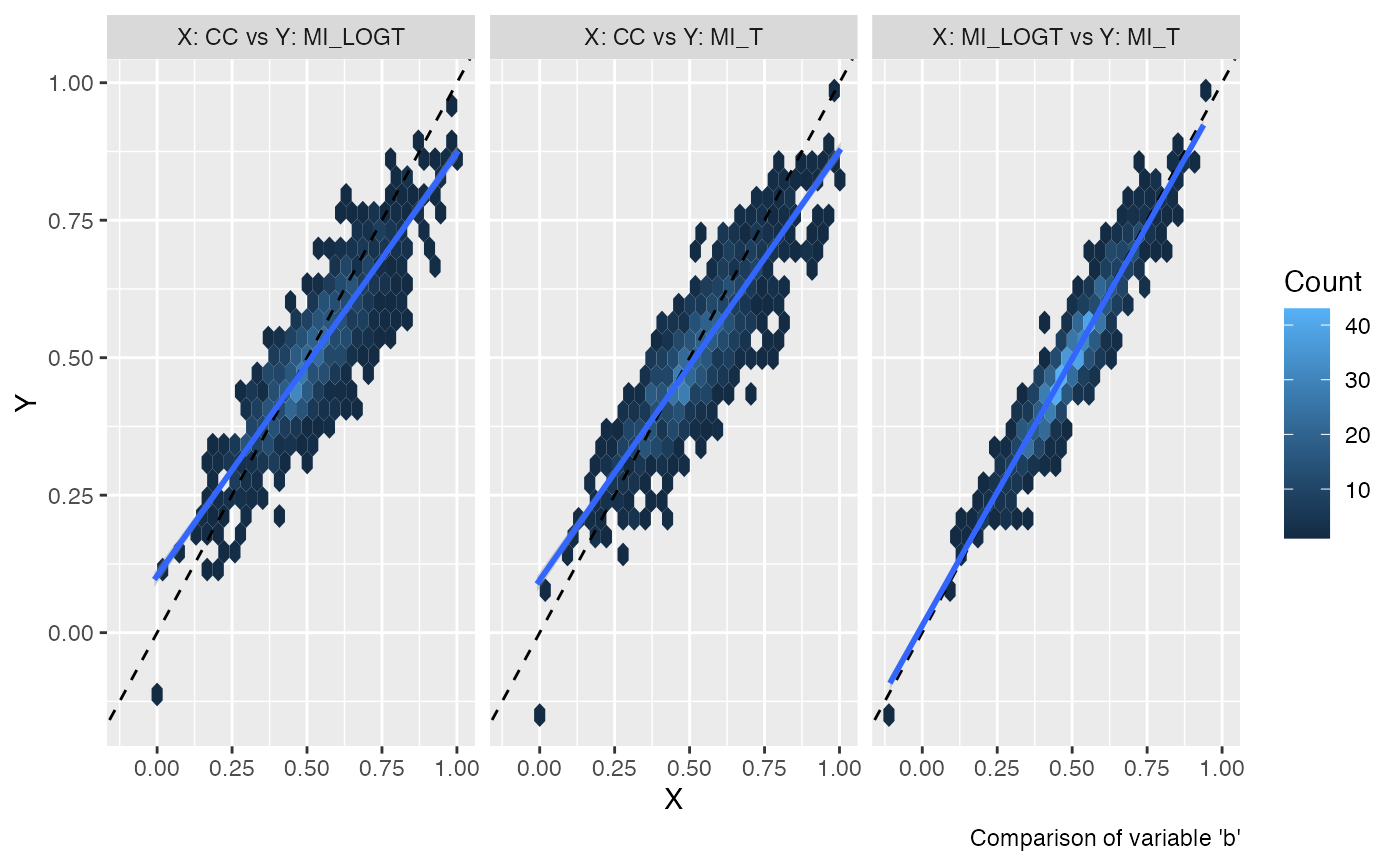autoplot(s, type = "zip", zoom = 0.5)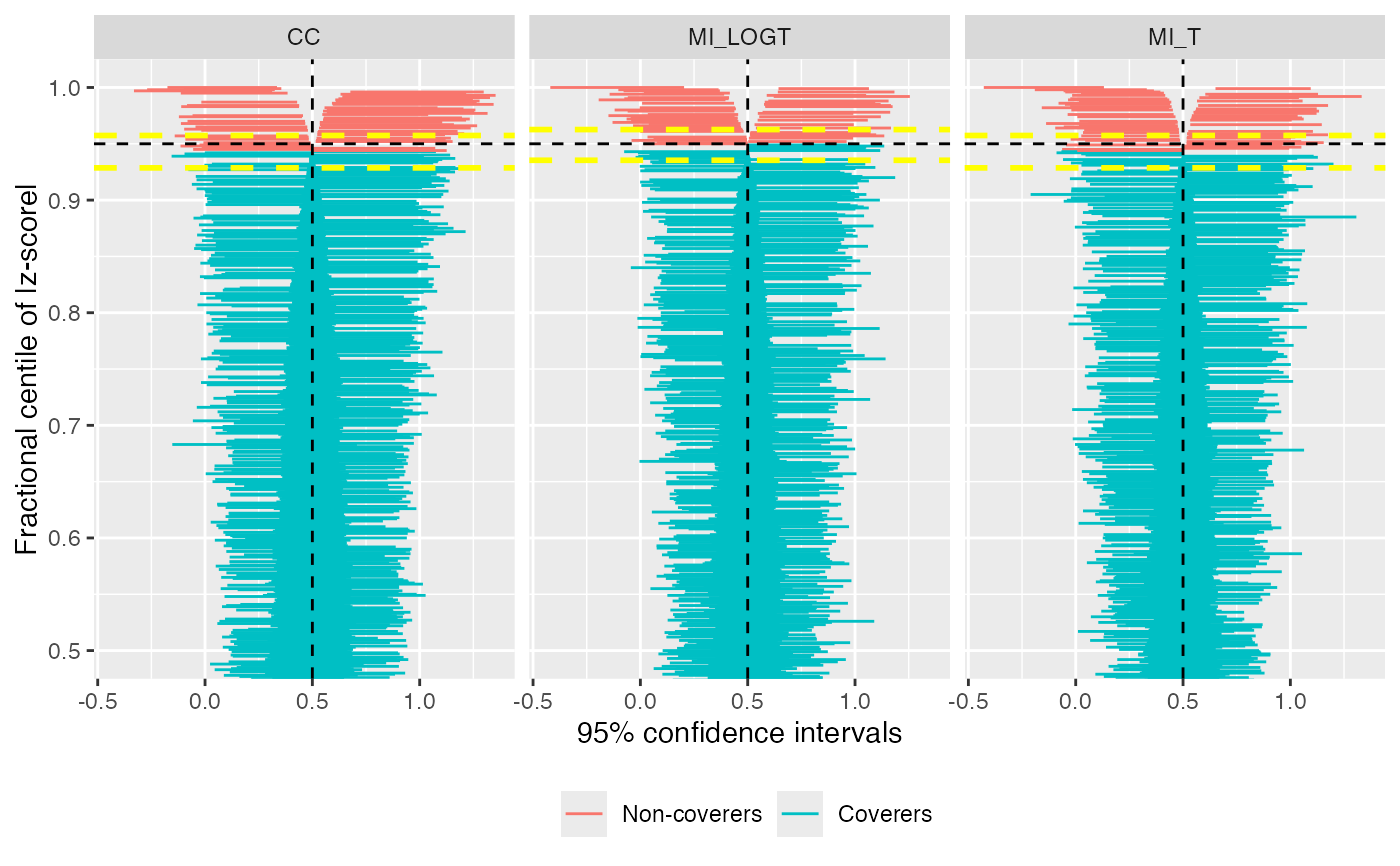# Nested loop plot:
data("nlp", package = "rsimsum")
s1 <- rsimsum::simsum(
data = nlp, estvarname = "b", true = 0, se = "se",
methodvar = "model", by = c("baseline", "ss", "esigma")
)#> 'ref' method was not specified, 1 set as the referenceautoplot(s1, stats = "bias", type = "nlp")